Search IntMath
Close

# How to Apply Powers to the Unit i

By Kathleen Cantor, 03 Apr 2021

The variablerepresents somewhat of an anomaly within mathematics. As one of the prerequisites to any type of non-real number, understanding i is a crucial step towards your journey into higher-level math. Before we apply powers to the unit, we must understand whatinvolves when we use it in a mathematical expression.

## What is?

As an imaginary number,doesn't fit into the realm of real numbers. It can't be calculated, nor can it interact with real numbers (outside of certain special cases). Commonly,is used as a stand-in for the imaginary value of the square root of -1.

However, the square root of -1 can't be calculated through real means. Still, we sometimes need its value to perform calculations using real numbers, like in the quadratic formula for example.

Certain mathematical expressions can only take specific types of input. As an example, the absolute value will only ever output positive numbers, while other expressions like square roots can't input or output negative values. These expressions are often referred to as non-negative functions.

## How Powers Affect the UnitAttempting to get a negative value out of these non-negative functions will output an error on any calculator. When it comes to using exponents, or powers, the same thing happens when you try to put any negative value into the function at all. Simply put, this is because performing the operation in that expression is impossible.

In a square root, there are no two negative numbers that are the same and can multiply together to create another negative number. Multiplying a negative number by a negative number always produces a positive one.

In these instances, we use the imaginary numberto get past the roadblock of square rooting a negative number and proceed with calculations.

### Performing Calculations Using the UnitIt's important to recognize whencan and cannot be used to perform calculations. A general rule of thumb is to treatas a variable that you can't evaluate. This means that most expressions containingcan be solved with simple algebra. Let's go over a few examples:

#### Example 1In this example, the expression cannot be evaluated. Be careful when working with both real and non-real numbers simultaneously - they exist in completely separate areas of mathematics! The equation above simply evaluates to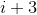.

#### Example 2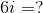In this case, we can't evaluate the expression for the same reason. A non-real number cannot interact with a real number outside of very specific circumstances. As a result, the expression still evaluates to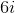.

It's important to be aware thatcan still have real numbers as its coefficient or divisor, even if the expression can't be further evaluated from that point. This is because attaching a coefficient or divisor todoesn't actually change the value that's denoted byin any way.

### Adding Powers to Your Equation withAlthough the following expression is still interacting with real numbers, this is one of the few special situations in which we can evaluate. Remember that, as a non-real number,is simply the stand-in for the square root of -1. Even though we can't evaluate the square root of -1, we can evaluate the square of a square root, which simply gives the value held within.

#### Example 1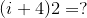In this instance, the square of i will yield a value of -1. With this information in mind, we can simplify the expression above:

••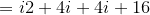•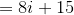Note that the distributive property still applies to non-real numbers, as we still are expanding two terms within brackets. The terms' actual content is irrelevant when it comes to the rules of expansion.

After applying distributive property, we are still left within the expression. But remember that non-real numbers cannot conventionally interact with i. As a result, after simplifying the expression's real numbers, we can no longer create any interactions between the real and non-real numbers.

Another important point is to recognize that expressions containingcan interact with each other and be simplified. Here, we were able to combine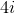andto create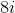, as bothandbelong to the non-real set of numbers. However, we cannot simplifyand 15 any further.

#### Example 2

In this example, we are given a cube of. Despite how it may first seem, the expression can be evaluated using exponent laws.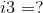We can rewrite the expression as follows: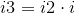By breaking the cube up intosquared multiplied by, it becomes clear that the expression can be evaluated using the same principle we used in the previous question. Reapplying our knowledge thatis a stand-in for the square root of 2 allows us to simplify the expression to the following: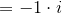At this point, we have a non-real number multiplied by a negative number. However, recall that we can still place coefficients and divisors before. As a result, the expression simplifies to: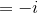Pretty straightforward, right?

## Final Takeaways

Essentially,is a variable that denotes the non-real number that would result from the square root of -1. It is most commonly used to further simplify expressions that otherwise would be unsimplifiable. Applying powers to the unitis fairly straightforward as long as you think ofas a variable with special properties and treat it that way.

Be the first to comment below.

### Comment Preview

HTML: You can use simple tags like <b>, <a href="...">, etc.

To enter math, you can can either:

1. Use simple calculator-like input in the following format (surround your math in backticks, or qq on tablet or phone):
a^2 = sqrt(b^2 + c^2)
(See more on ASCIIMath syntax); or
2. Use simple LaTeX in the following format. Surround your math with $$ and $$.
$$\int g dx = \sqrt{\frac{a}{b}}$$
(This is standard simple LaTeX.)

NOTE: You can mix both types of math entry in your comment.